# Revisiting the Register File

If you happen to be one of the two readers of this blog who have actually checked out the circuits I’ve posted, you might have found out that the register file circuit (RF) I presented in an earlier post doesn’t work quite right. If you tried it out by manually changing the input values (e.g. `rD` and `dD`), everything appears to work correctly. Whenever the clock ticks, the new value is written to the selected register.

The problem I’m going to talk about appears when you try to use this component as part of a larger circuit. If you connect your clock directly to the `clk` input of the RF but the `dD` and `rD` inputs are connected to some other component’s output, you’ll need 2 clock ticks to actually write the new value to the selected register. This is because I didn’t pay attention to propagation delays when designing the various components and the clock signal arrives to the flip flops before the new data signal.

So, let’s fix the circuit by starting from the basic element of the RF, as I did in the original post.

## Positive edge triggered D flip flop

The original DFF I used is shown in figure 1 for reference. Ideally, whenever `clk` goes from LOW to HIGH, the current `D` value is reflected on the `Q` output. Unfortunately, this isn’t true. In order to test it out we need a testbench. Since `D` is an 1-bit signal, there are two transitions to consider: `D` going from LOW to HIGH and vice versa.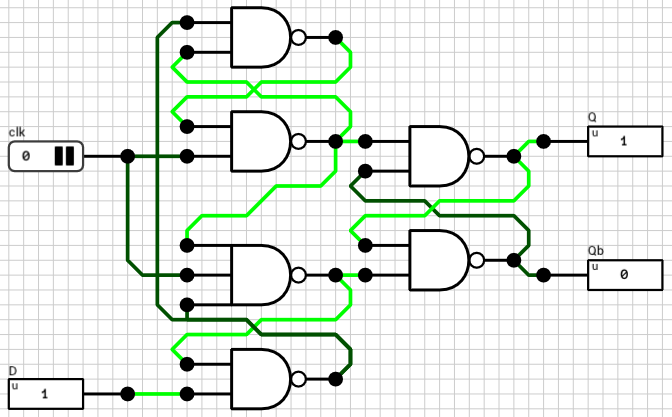Figure 1: The original Positive Edge Triggered D Flip Flop circuit

There are 3 different cases when it comes to the timings of `clk` and `D` inputs.

1. `clk` signal arrives before `D`
2. `clk` signal arrives at the same time as `D`
3. `clk` signal arrives after `D`

Cases 2 and 3 are the ones we are interested in. The 1st case works correctly because if the clock signal arrives before the new data, it means that the controlling circuit wanted to write the old data to the flip flop. In other words, it’s the controlling circuit’s responsibility to synchronize the two signals.

On the other hand, if the rising edge of `clk` arrives after the new `D` value, we must assume that the new data will be written to the flip flop. So, the worst case scenario is that both `clk` and `D` arrive at the exact same time.

In order to find out if the current circuit works as expected, I used a testbench. Testbenches are an easy way to change multiple input values at the same time before triggering a simulation. Script 1 below shows the testbench I used.

``````-- Reset the circuit to a known state
set("clk", 0);
set("D", 0);
simulate();

-- D 0 -> 1
set("D", 1);
tick("clk");
assert(get("Q") == 1, "Failed");
assert(get("Qb") == 0, "Unstable!");
tick("clk");

-- D 1 -> 0
set("D", 0);
tick("clk");
assert(get("Q") == 0, "Failed");
assert(get("Qb") == 1, "Unstable!");
tick("clk");
``````

Script 1: DFF testbench

Initially the circuit is reset to a known state (`clk = 0` and `D = 0`). The first test is for the LOW-to-HIGH `D` transition and the second and final test is for the HIGH-to-LOW transition. Remember that `tick()` toggles the specified clock value and triggers a simulation.

If you execute this testbench in the simulator you’ll find out that the HIGH-to-LOW transition of the `D` signal doesn’t work (the first assert of the second test is triggered and the testbench is terminated). This means that when `D` goes from HIGH to LOW, the time required for the `clk` signal to arrive to the output latch is less than the time required for the new `D` value, which results in the old `D` value being written to it.

In order to fix it, the clock signal should be delayed. The easiest way to delay a signal in the current version of DLS is to use an AND gate. By passing the same signal to all its inputs, you get the same value on its output, at a later (internal) timestep. In DLS, each basic gate has its own propagation delay, which is dependent on the number of inputs (check appendix A of the manual for details). In our case, an AND2 gate has a delay of 1T and an AND3 gate has a delay of 2T.

By trial and error, I found that the required delay for the clock signal is 2T (a single AND3 gate or two AND2 gates in series). The final, corrected, DFF circuit is shown in figure 2.Figure 2: The corrected Positive Edge Triggered D Flip Flop circuit

The testbench works correctly with this circuit. This means that if the controlling circuit sends both signals at the exact same timestep, the flip flop will work correctly. If the clock signal arrives at a later timestep than the `D` signal it will also, by definition, work correctly.

## 1-bit Register

In the same vein, let’s test the original 1-bit register (figure 3). In this case, there’s an extra 1-bit input (`load`) which determines if the new `D` value will be written to the flip flop or not. The testbench used to check this circuit is shown in Script 2.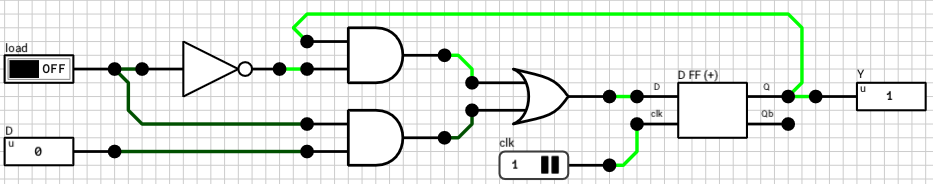Figure 3: The original 1-bit Register
``````-- Reset circuit
set("clk", 0);
set("Din", 0);
simulate();

-- D: 0 -> 1, load: 1
set("Din", 1);
tick("clk");
assert(get("Dout") == 1, "Failed");
tick("clk");

-- D: 1 -> 0, load: 1
set("Din", 0);
tick("clk");
assert(get("Dout") == 0, "Failed");
tick("clk");

-- D: 0 -> 1, load: 1 -> 0
set("Din", 1);
tick("clk");
assert(get("Dout") == 0, "Failed");
tick("clk");

-- D: 1, load: 0 -> 1
tick("clk");
assert(get("Dout") == 1, "Failed");
tick("clk");

-- D: 1 ->, load: 1 -> 0
set("Din", 0);
tick("clk");
assert(get("Dout") == 1, "Failed");
tick("clk");
``````

Script 2: 1-bit Register testbench

The delay of the critical path of the DFF controlling circuit (i.e. the multiplexer in front of the DFF) is 3T (from `load` to OR output). So in order to make `D` and `clk` arrive at the same time to the DFF component, the clock should be delayed by 3T (one AND2 gate and one AND3 gate in series). Figure 4 shows the new 1-bit register circuit which passes all the tests in the testbench.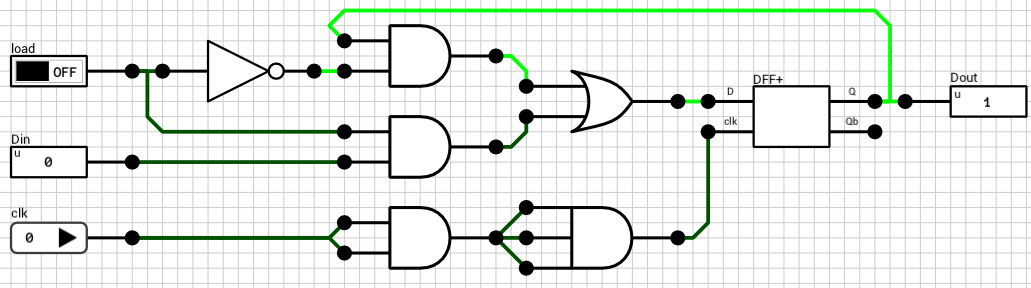Figure 4: The corrected 1-bit Register circuit

## 16-bit Register

Once more, the clock in the 16-bit register circuit should be delayed until the `D` signal is ready to be fed to the 1-bit registers. Only a 16-bit wire splitter exists between `Din` and the 16 1-bit registers and the wire splitter has a delay of 1T (independent of the number of bits). So by delaying the clock signal by 1T, both `Din` and `clk` arrive at the 1-bit registers at the same time. Note that the `load` signal is already split and directly connected to the registers, so it should be valid when `clk` and `Din` arrive.

Script 3 below shows the testbench for the final 16-bit register circuit from figure 5. This time, since the number of possible transistions of the `Din` signal are way too many to exhaust, I used random inputs for the `Din` port.

``````set("clk", 0);
set("Din", 0);
simulate();

local D = randBits(16);
set("Din", D);
tick("clk");
assert(get("Dout") == D, "Failed");
tick("clk");

for i=1, 1000 do
local v = randBits(16);
local load = randBits(2);

set("Din", v);

tick("clk");

local expectedValue = v;
if(load == 0) then
expectedValue = D;
elseif(load == 1) then
local low = bit.band(v, 0x00FF);
local high = bit.band(D, 0xFF00);
expectedValue = bit.bor(low, high);
elseif(load == 2) then
local low = bit.band(D, 0x00FF);
local high = bit.band(v, 0xFF00);
expectedValue = bit.bor(low, high);
else
expectedValue = v;
end

assert(get("Dout") == expectedValue, "Failed");

D = expectedValue;

tick("clk");
end
``````

Script 3: 16-bit Register testbench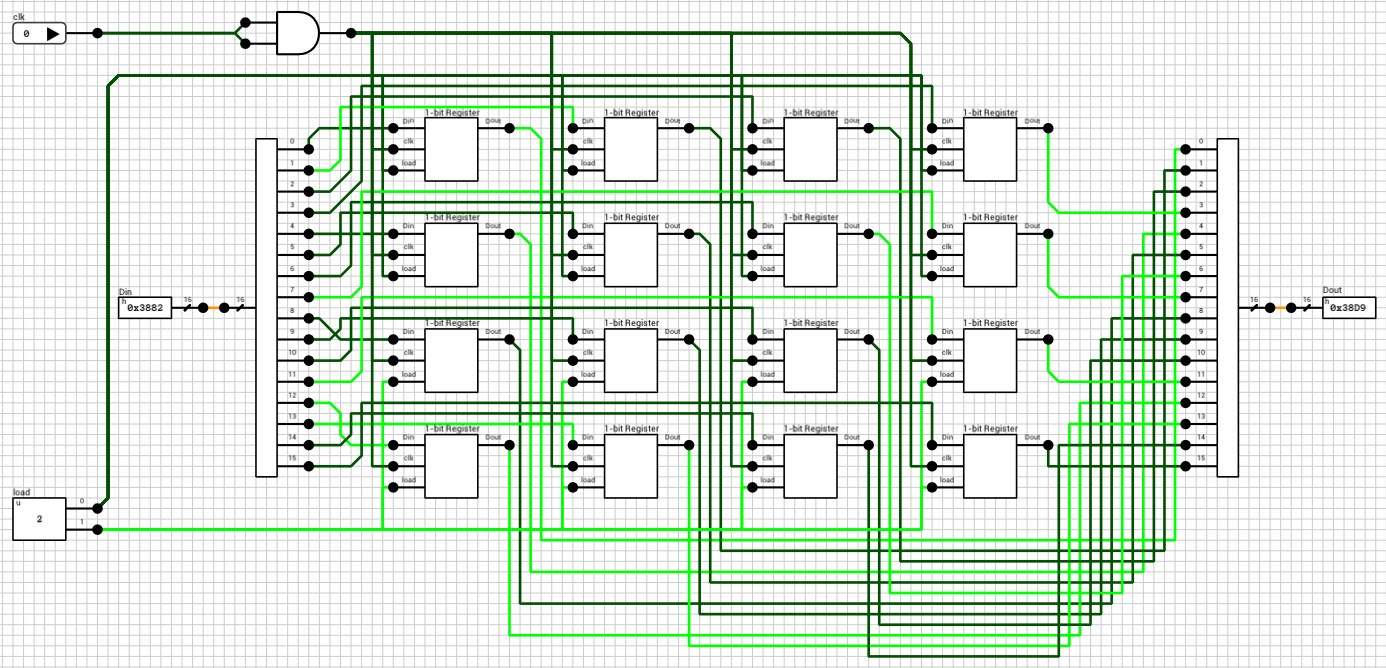Figure 5: The corrected 16-bit Register circuit

## 8x16-bit Register file

Finally it’s time to look the actual register file circuit (figure 6). We are only interested in the write part of the circuit, since reading is performed asynchronously (whenever `rA`, `rB`, `oeA` or `oeB` change, the outputs are immediately updated, without waiting for a `clk` rising edge).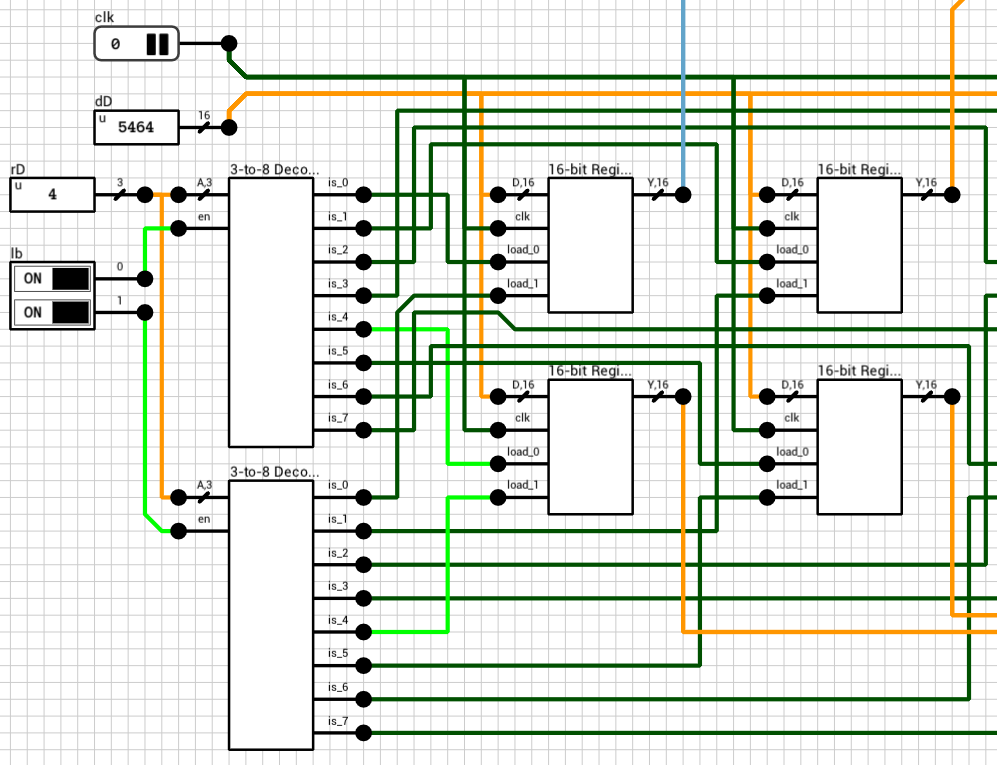Figure 6: The original 8x16-bit Register File circuit (write part)

Both `dD` and `clk` are directly connected to the corresponding inputs of all 8 registers so it’s probably expected that the circuit will work correctly once we replace the old registers with the new components presented above. Script 4 shows a small testbench.

``````-- Reset the circuit. Don't touch dD for extra randomness :)
set("rA", 0);
set("rB", 1);
set("rD", 0);
set("lb", 3); -- Write both bytes to simplify testing
set("clk", 0);
simulate();

-- Test 1: Write a random value to register 0.
local v = randBits(16);
set("dD", v);
tick("clk");
assert(get("dA") == v, "Failed");
tick("clk");

-- Test 2: Write a random value to register 1.
local v2 = randBits(16);
set("dD", v2);
set("rD", 1);
tick("clk");
assert(get("dA") == v, "Failed");
assert(get("dB") == v2, "Failed");
tick("clk");
``````

Script 4: 8x16-bit Register File testbench

As always, the circuit is first reset to a known state. `rA` and `rB` are pointed to registers 0 and 1 respectively, `rD` (the destination register) is set to 0 and `lb` is set to 3, meaning both bytes will be written, to simplify testing.

Test 1 tries to write a new random value to register 0. What’s expected is that when `clk` rises, the new value should be written to the register and `dA` should be updated to reflect it. This works correctly, since the registers have been corrected to handle both signals arriving at the same time.

The 2nd test tries to write another random value to register 1, by switching `rD` to 1 and `dD` to the new value, at the same timestep. It’s expected that when `clk` rises, the new value should be written to the register and `dB` should be updated to reflect it. Unfortunately, this part doesn’t work correctly!

The reason is that there’s a delay on the `load` inputs of each register. By the time `clk` and `dD` arrive at the registers, the old `rD` is used to select the destination, because the 3-to-8 decoder haven’t had a chance to calculate its new output yet.

Looking at the 3-to-8 decoder (figure 7), the critical path delay is 4T, from `A` to `is` (1T for the wire splitter, 1T for the NOT gates and 2T for the AND4 gates). So, delaying the `clk` signal by 4T should do the trick.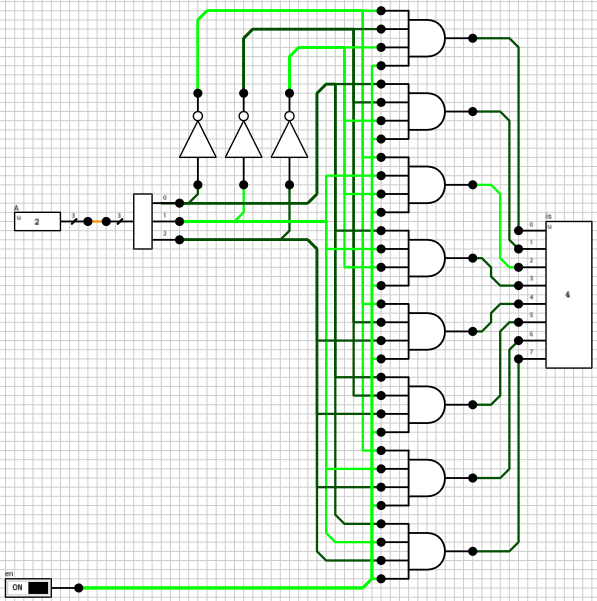Figure 7: Gated 3-to-8 decoder circuit

Figure 8 shows the final register file circuit. The 4T delay has been added to the `clk` signal using two AND3 gates.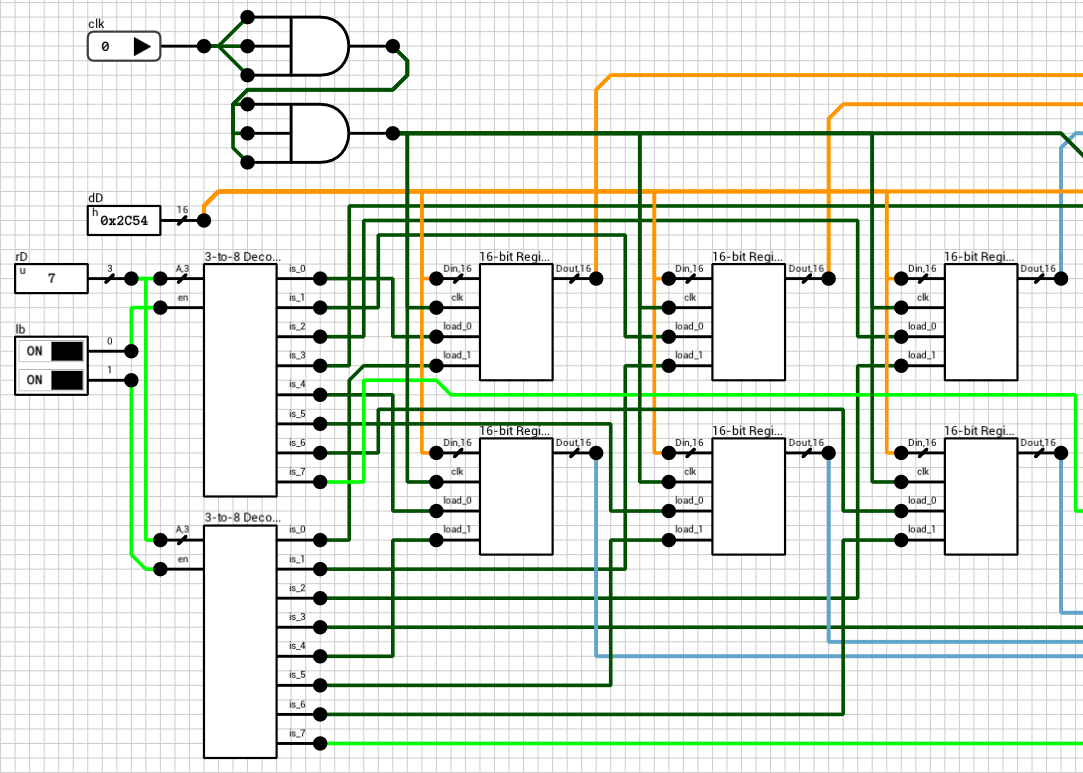Figure 8: The corrected 8x16-bit Register File circuit (write part)

## Conclusion

If there’s something to keep in mind from this post is that whenever there’s a register/flip flop in a circuit, you should make sure that clock’s rising or falling edge arrives to it at the same time or after the data signal. Otherwise, you might need an extra clock cycle to actually store the new value in the register.

Note that the old version worked correctly in all other aspects. It just needed an extra rising edge to actually write the new value to the registers, which sometimes might be annoying when trying to debug it. Having the register file behave in the way we did in this post will make things a bit easier to debug when this component is used in a larger circuit.

Until the next post, comments/suggestions/corrections are welcome.